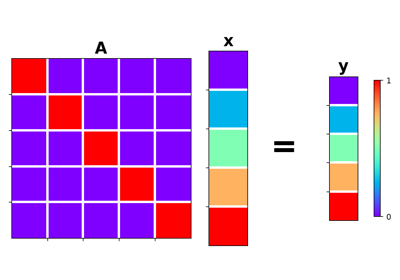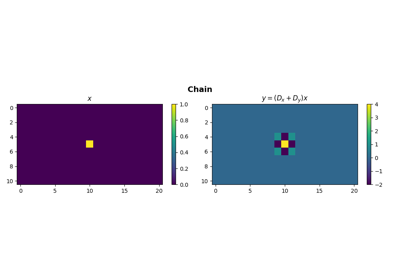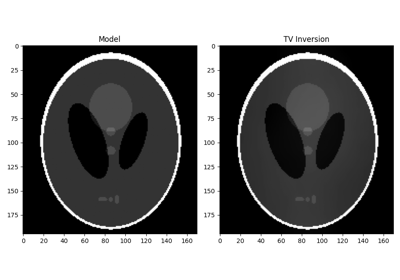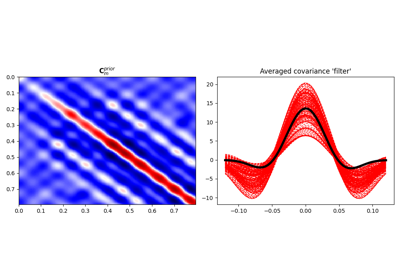# pylops.Identity#

class pylops.Identity(N, M=None, inplace=True, dtype='float64', name='I')[source]#

Identity operator.

Simply move model to data in forward model and viceversa in adjoint mode if $$M = N$$. If $$M > N$$ removes last $$M - N$$ elements from model in forward and pads with $$0$$ in adjoint. If $$N > M$$ removes last $$N - M$$ elements from data in adjoint and pads with $$0$$ in forward.

Parameters
Nint

Number of samples in data (and model, if M is not provided).

Mint, optional

Number of samples in model.

inplacebool, optional

Work inplace (True) or make a new copy (False). By default, data is a reference to the model (in forward) and model is a reference to the data (in adjoint).

dtypestr, optional

Type of elements in input array.

namestr, optional

New in version 2.0.0.

Name of operator (to be used by pylops.utils.describe.describe)

Notes

For $$M = N$$, an Identity operator simply moves the model $$\mathbf{x}$$ to the data $$\mathbf{y}$$ in forward mode and viceversa in adjoint mode:

$y_i = x_i \quad \forall i=1,2,\ldots,N$

or in matrix form:

$\mathbf{y} = \mathbf{I} \mathbf{x} = \mathbf{x}$

and

$\mathbf{x} = \mathbf{I} \mathbf{y} = \mathbf{y}$

For $$M > N$$, the Identity operator takes the first $$M$$ elements of the model $$\mathbf{x}$$ into the data $$\mathbf{y}$$ in forward mode

$y_i = x_i \quad \forall i=1,2,\ldots,N$

and all the elements of the data $$\mathbf{y}$$ into the first $$M$$ elements of model in adjoint mode (other elements are O):

\begin{align}\begin{aligned}x_i = y_i \quad \forall i=1,2,\ldots,M\\x_i = 0 \quad \forall i=M+1,\ldots,N\end{aligned}\end{align}
Attributes
shapetuple

Operator shape

explicitbool

Operator contains a matrix that can be solved explicitly (True) or not (False)

Methods

 __init__(N[, M, inplace, dtype, name]) adjoint() apply_columns(cols) Apply subset of columns of operator cond([uselobpcg]) Condition number of linear operator. conj() Complex conjugate operator div(y[, niter, densesolver]) Solve the linear problem $$\mathbf{y}=\mathbf{A}\mathbf{x}$$. dot(x) Matrix-matrix or matrix-vector multiplication. eigs([neigs, symmetric, niter, uselobpcg]) Most significant eigenvalues of linear operator. matmat(X) Matrix-matrix multiplication. matvec(x) Matrix-vector multiplication. reset_count() Reset counters rmatmat(X) Matrix-matrix multiplication. rmatvec(x) Adjoint matrix-vector multiplication. todense([backend]) Return dense matrix. toimag([forw, adj]) Imag operator toreal([forw, adj]) Real operator tosparse() Return sparse matrix. trace([neval, method, backend]) Trace of linear operator. transpose()

## Examples using pylops.Identity#Identity

IdentityOperators concatenation

Operators concatenationTotal Variation (TV) Regularization

Total Variation (TV) Regularization04. Bayesian Inversion

04. Bayesian Inversion